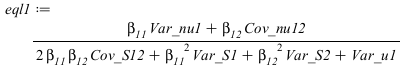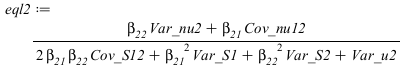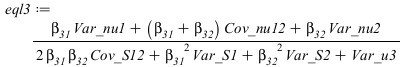## dharr

Dr. David Harrington

## 4791 Reputation

19 years, 34 days
University of Victoria
Professor or university staff

## Social Networks and Content at Maplesoft.comI am a professor of chemistry at the University of Victoria, BC, Canada, where my research areas are electrochemistry and surface science. I have been a user of Maple since about 1990.

## Tarjan...

@lcz Tarjan's algorithm seems to be standard, and was for directed graphs, (but works also for undirected if allowance is made for the double counting). So, yes it is strange not to have the capability for both.

It was the fastest of the algorithms I played with, but perhaps with dense graphs other algorithms might be better. Tarjans's could probably be converted to a compiled version in Maple, if the stack was implemented "by hand" using arrays. I was originally thinking algorithms based on cycle basis was the way to go, but the literature doesn't seem to point to that.

## Zeon algebra - just counts...

I also have another version that uses Zeon algebra, but just counts and doesn't produce the cycles themselves, so I added it to the other thread:

How-To-Determine-The-Number-Of-Cycles-Of--Graph

Infolevel gives information that is not that enlightening.

solve.mw

I can't decide whether this is a bug, or whether we are expected to be cautious when mathematical functions like ln are involved.

## documentation...

@lcz ?GraphTheory,Details for the documentation. op(4,G) is the Array that is very similar to what Neighbor returns, though Neighbors uses the names of the Vertices rather than the numbers.

In fact, since almost all (all?) other GraphTheory commands return vertices as names, it seems like an oversight (bug?) that IsChordal returns the numbers of the vertices. But it is so poorly documented it is hard to tell what was intended.

## slightly different...

@Carl Love I wasn't suggesting the stop button as a solution to those longer term issues. The OP's description suggested that they lost worksheets when this hangs, which suggests that their system is not responding to the stop button, or the exit application button (which often gives an oppportunity to save even if Maple has ceased responding). If that is the case, then my comment might suggest that there is some system difference that technical support might solve.

## matrix formulation...

@MaPal93 The quintic has all coefficients positive, except for the constant term, which is negative. So if you removed the constant term and plotted it, it goes through the origin and then is monotonically increasing as x increases. Adding the (negative) constant term brings the curve down, and will mean there is exactly one positive root. (Sturm sequences tell something about location of real roots in the more general case; Maple has them only for constant coefficients, but you could apply them for the general case, though I'm not sure this will do much better than solve( , parametric) or the other solvers with inequalities.)

I am trying to formulate your three equations as much as possible in matrix form. Of course you probably got them from some matrices in the first place so I am probably just reverse engineering here. I don't know much about statistics but am reasonably fluent with matrices. I see the matrix origin of the betas. Then in your equations:the matrix origins, if any, are much harder to see. Would you mind answering some questions that might help me with this?

1. In these equations you introduce new variables Cov_S12, Var_S1, Var_S2, Var_nu1, Var_nu2, Cov_nu12 - is there any relationship between these and the earlier variables?

2. You also have the variables Var_u1, Var_u2, Var_u3 that were used previously. In the earlier equations that you solved, these (and all the other variances and covariances) got multiplied by gamma. Should these also be multiplied by gamma here? Or perhaps gamma multiplied the other new variables as well and then cancelled in these equations?

3. Is theta__12 = theta__21?

4. Since beta__12 is not the same as beta__21, 2*beta__11*beta__12*Cov_S12 is not the same as beta__11*(beta__12+beta__21)*Cov_S12. The latter form seems more natural for a matrix formulation. Is it correct as written or is there perhaps a hidden assumption here?

Here are my manipulations so far:  Matrix4.mw

If you think this is a profitable approach, I'd be happy to elaborate on this.

Some responses:

"Your code is not using dsolve numeric in parametric form. I will be posting the use of parametric form working with NLPSolve (thanks to Allan Wittkopf)."

I agree - as I said, I thought from your original post you may have been looking for a workaround.

As I said, we don't want analytical solutions.

This was just to test my answer.

Also, one parameter optimization is different from multiple parameters (scalar vs vector).

Sorry, I have misunderstood the problem statement. In your reply to @vv you said

"The problem statement is given below

dca/dt = -(u+u^2/2)*ca

dcb/dt = u*ca - 0.1*cb

t = 0, ca = 1, cb = 0
Maximize cb(1) subject to the bounds 0 < u< 5."

which seemed to me to have only one parameter.

My method is easily extended to more parameters.

To be clear, I am not looking for an answer for the model. My goal was to use NLPSolve with dsolve/numeric/parameters with sqp.

Yes, I understood that was just a test problem, and I was proposing a generic solution. In view of your comments about the complexity of the solution for least squares, I stripped done my more general earlier answer to make it more clear how it works.

But anyway it seems you now have a solution. Good luck!

@ The code is that way because I had it already at hand, and I didn't have a lot of time this morning. The complexity of the code was to encapsulate the whole thing in a user-friendly callable routine without using global variables. I realize that was not your issue, but since you were having problems with the dsolve parametric form, I thought you might be interested in this alternative way of doing it, which just substitutes the parameters in.

It is still easier for me to rewrite than debug your code. I'm guessing somehow the nvars=2 problem just doesn't have enough degrees of freedom to be sensibly done by NLPSolve, since it works with nvars higher. Anyway, here is a solution to your problem if I have understood it correctly. If you need the function cb at various specified times, you can use the output=Array option in the final dsolve call.

I went back to the procedure form, since then you can let the dsolve error mechanism decide on the stepsize etc and bypass using nvar. In my experience the Matrix method isn't much faster, but that depends on the class of problems.

 > restart;

VS problem - for the solution of the following ode system with parameter u, maximize cb(1) using NLPSolve with method=sqp and bounds =0..5

 > des:={diff(ca(t),t)=-(u+u^2/2)*ca(t),diff(cb(t),t)=u*ca(t)-(1/10)*cb(t),ca(0)=1,cb(0)=0};Analytical solution for comparison

 > ans:=dsolve(des);> cb1:=eval(eval(cb(t),ans),t=1); umax:=fsolve(diff(cb1,u)=0,u=0..2); cb1max:=evalf(eval(cb1,u=umax));> plot(cb1, u = 0 .. 5,color=red);> SS:=proc(uu)     local ans;     if not type(uu,numeric) then return 'procname'(args) end if;       ans:=dsolve(eval(des,u=uu),{ca(t),cb(t)},numeric);     eval(cb(t),ans(1));   end proc:
 > opt:=Optimization:-NLPSolve(SS,0..5,initialpoint=,method=sqp,maximize=true);> ufound:=dsolve(eval(des, u = opt), {ca(t), cb(t)}, numeric);> plots:-odeplot(ufound,[t,cb(t)],0..5);>## sqp...

I now tried it with sqp, and it seems to work, but is slower.

## numerical issue...

@MaPal93 It turns out that it is a solution, one just needs more accuracy to show that:

Numerical_issue.mw

The small change in length I think just means Maple generated and stored the expression in a slightly different form.

## problem with rho__u case...

@MaPal93 The rho_u case seems to have a positive solution, but when I substitute into EqN_rhou I don't find it is a solution. Perhaps the solution matches another version of EqN_rhou?

250523_SOLUTION_analytical2.mw

## assumptions...

@MaPal93

"Does it mean that the quadratic solutions I obtain from the solver would be the same regardless of the assumptions on my variables and parameters (and only the roots to these quadratic equations depend on the assumptions)?"

Yes, I do not think PolynomialSystem is using the assumptions and so you could (should) leave them out. In principle if you put them in, say by using RegularChains:-Triangularize directly (where you can just add them to the set of equations), and it could figure out that the quadratic did not have any positive solutions then it would not return the quadratic as a solution. I only tried RegularChains:-Triangularize once with inequalities and they seemed to slow it down so much I didn't wait for a solution, on a problem smaller than yours.

## assumptions...

@MaPal93 In general, Maple doesn't handle assumptions particularly well, so I usually leave them out, or just add "assuming" clauses when I really need them. For solve itself, you need "useassumptions" as a solve option for the assuming clause to take effect - see the gamma file I uploaded for an example. For the polynomial systems if you look at RegularChains:-Triangularize that PolynomialSysyem is using, you can see how to more specifically add inequalities, but it might be faster to process them after as needed.

@MaPal93 Because a set cannot have duplicates, if you do a substitution that reduces nops from 3 to 2, then two of these equations became equal. (To see which are equal you could do the substitution on the individual equations and then compare them, by seeing if simplification of their differences was zero.)

I analysed the quadratic solution of the gamma equation, and found there are no all-positive solutions.

Edit: forgot the file:

220523_SOLUTION_reducedform_calibrations2.mw

## easy to solve if lambda__2 = lambda__1...

@dharr the positive solution had lambda__1 = lambda__2 (some others did too). If there is some reason why this will be always true, then the system of 3 equations reduces to two, and can be solved extremely quickly with solve.

EqN2.mw

﻿